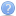Latex symbol
It is not that big a problem. It is only a minor inconvenience. Thank you for spending the time to look into this and letting me know. Thanks for all your hard work on Textastic.Latex symbol
Here is a part of the LaTeX document that I use.

\documentclass[12pt]{report}

\usepackage{amsthm}            % Introduces theorems

% Header and footer for when a page split occurs within a problem environment
\nobreak\extramarks{#1}{#1 continued on next page\ldots}\nobreak
\nobreak\extramarks{#1 (continued)}{#1 continued on next page\ldots}\nobreak
}

% Header and footer for when a page split occurs between problem environments
\nobreak\extramarks{#1 (continued)}{#1 continued on next page\ldots}\nobreak
\nobreak\extramarks{#1}{}\nobreak
}

\setcounter{secnumdepth}{0}         % Removes default section numbers
\newcounter{homeworkProblemCounter} % Creates a counter to keep track of the number of problems

\newcommand{\homeworkProblemName}{}
\newenvironment{homeworkProblem}[Problem \arabic{homeworkProblemCounter}]{
% Makes a new environment called homeworkProblem which takes 1 argument (custom name) but the default is "Problem #"
\stepcounter{homeworkProblemCounter}    % Increase counter for number of problems
\renewcommand{\homeworkProblemName}{#1} % Assign \homeworkProblemName the name of the problem

\section{\homeworkProblemName} % Make a section in the document with the custom problem count

}{
}

% Defines the problem answer command with the content as the only argument
\noindent\framebox[\columnwidth][c]{\begin{minipage}{0.98\columnwidth}#1\end{minipage}}
% Makes the box around the problem answer and puts the content inside
}

\newcommand{\homeworkSectionName}{}
\newenvironment{homeworkSection}{
% New environment for sections within homework problems, takes 1 argument - the name of the section
% Assign \homeworkSectionName to the name of the section from the environment argument
\renewcommand{\homeworkSectionName}{#1}
\subsection{\homeworkSectionName}        % Make a subsection with the custom name of the subsection
}{
}
\begin{document}
\begin{homeworkProblem}
Sample Text Here
\begin{homeworkSection}{NameSection}
More text
\end{homeworkSection}
other text
\end{homeworkProblem}

\begin{homeworkProblem}
\begin{thrm}{}
\end{thrm}
\begin{proof}
\end{proof}
\end{homeworkProblem}

\end{document}


This is mostly from templates I have found and modified a small portion. Anyways in textastic (the desktop or mobel version) in the symbol list I only see two symbols one is \homeworkProblemName and if clicked it reffers to the line inside the \newenvironment definition for homeworkProblem that creates the new section.

\newenvironment{homeworkProblem}[Problem \arabic{homeworkProblemCounter}]{
...
\section{\homeworkProblemName} % This line
...
}


Similarly there is another symbol in the symbol list \homeworkSectionName which is marked as a subsection and when clicked, I am referred to the line in the \newenvironment definition for homeworkSection that creates the subsection.

\newenvironment{homeworkSection}{
...
\subsection{\homeworkSectionName}        % This line
...
}


﻿